### Playing with Numbers - Solutions 2

CBSE Class –VIII Mathematics
NCERT Solutions
CHAPTER - 16
Playing with Numbers
(Ex. 16.2)

1. If 21y5 is a multiple of 9, where is a digit, what is the value of y?
Ans. Since 21y5 is a multiple of 9.
Therefore according to the divisibility rule of 9, the sum of all the digits should be a multiple of 9.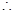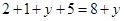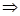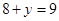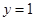Since 21y5 is a multiple of 9.

2. If 31z is a multiple of 9, where z is a digit, what is the value of z? You will find that there are two answers for the last problem. Why is this so?
Ans.  Since 31z5 is a multiple of 9.
Therefore according to the divisibility rule of 9, the sum of all the digits should be a multiple of 9.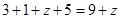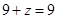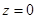If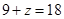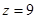Hence 0 and 9 are two possible answers.

3. If 24is a multiple of 3, where is a digit, what is the value of x?
(Since 24is a multiple of 3, its sum of digits 6 + is a multiple of 3; so 6 + is one of these numbers: 0, 3, 6, 9, 12, 15, 18, ... .But since is a digit, it can only be that
6 + = 6 or 9 or 12 or 15. Therefore, = 0 or 3 or 6 or 9. Thus, can have any of (four different values.)
Ans.  Since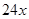is a multiple of 3.
Therefore according to the divisibility rule of 3, the sum of all the digits should be a multiple of 3.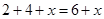Since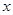is a digit.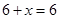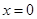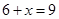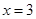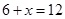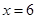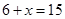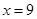Thus,can have any of four different values.

4.     If 31z5 is a multiple of 3, where z is a digit, what might be the values of z?
Ans. Since 31z5 is a multiple of 3.
Therefore according to the divisibility rule of 3, the sum of all the digits should be a multiple of 3.
Since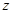is a digit.If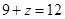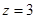If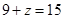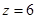IfHence 0, 3, 6 and 9 are four possible answers.